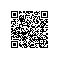# 好程序员Python教程系列-第6讲：循环结构

好程序员Python教程系列-第6讲：循环结构，应用场景：我们在写程序的时候，一定会遇到需要重复执行某条或某些指令的场景。例如用程序控制机器人踢足球，如果机器人持球而且还没有进入射门范围，那么我们就要一直发出让机器人向球门方向移动的指令。在这个场景中，让机器人向球门方向移动就是一个需要重复的动作，当然这里还会用到上一课讲的分支结构来判断机器人是否持球以及是否进入射门范围。再举一个简单的例子，如果要实现每隔1秒中在屏幕上打印一次“hello, world”并持续打印一个小时，我们肯定不能够直接把print('hello, world')这句代码写3600遍，这里同样需要循环结构。

for-in循环

"""

Version: 0.1
Author: 骆昊
"""
total = 0
for x in range(1, 101):

total += x

print(total)

range(101)：可以用来产生0到100范围的整数，需要注意的是取不到101。
range(1, 101)：可以用来产生1到100范围的整数，相当于前面是闭区间后面是开区间。
range(1, 101, 2)：可以用来产生1到100的奇数，其中2是步长，即每次数值递增的值。
range(100, 0, -2)：可以用来产生100到1的偶数，其中-2是步长，即每次数字递减的值。

"""

Version: 0.1
Author: 骆昊
"""
total = 0
for x in range(2, 101, 2):

total += x

print(total)
while循环

"""

Version: 0.1
Author: 骆昊
"""
import random

counter = 0
while True:

counter += 1
number = int(input('请输入: '))
print('大一点')
print('小一点')
else:
print('恭喜你猜对了!')
break

print(f'你总共猜了{counter}次')
break和continue

"""

Version: 0.1
Author: 骆昊
"""
for i in range(1, 10):

for j in range(1, i + 1):
print(f'{i}*{j}={i * j}', end='\t')
print()

"""

Version: 0.1
Author: 骆昊
"""
num = int(input('请输入一个正整数: '))
end = int(num ** 0.5)
is_prime = True
for x in range(2, end + 1):

if num % x == 0:
is_prime = False
break

if is_prime and num != 1:

print(f'{num}是素数')

else:

print(f'{num}不是素数')

"""

Version: 0.1
Author: 骆昊
"""

x = int(input('x = '))
y = int(input('y = '))
if x > y:

x, y = y, x    # Python中可以用这样的方式来交换两个变量的值

for factor in range(x, 0, -1):

if x % factor == 0 and y % factor == 0:
print(f'{x}和{y}的最大公约数是{factor}')
print(f'{x}和{y}的最小公倍数是{x * y // factor}')
break使用钉钉扫一扫加入圈子
+ 订阅